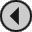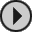## 3D CONSTRUCTION KIT

### Two Byte Values (0-65535)

If, for example we set variable 10 to hold the game score, for display via an Instrument as a 5 character number. Two variables (bytes) are required to store this. Variable 10 stores the LOW byte (first) and variable 11 stores the HIGH byte (last). To add 100 to the score the following would be performed:
```   ADDV 100 10

(Add the carry, if there is one, to variable 11)
```
To add 300 to the score the 300 must be broken down to two bytes thus:
300 divided by 256 = 1 remainder 44
So to add 300 to our score:
```   ADDV  44 10

```   SUBV  44 10